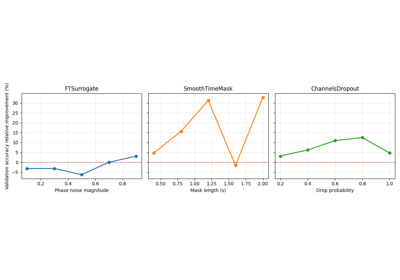Smoothly replace a randomly chosen contiguous part of all channels by zeros.

Suggested e.g. in  and 

Parameters
• probability (float) – Float setting the probability of applying the operation.

• mask_len_samples (int | torch.Tensor, optional) – Number of consecutive samples to zero out. Will be ignored if magnitude is not set to None. Defaults to 100.

• random_state (int | numpy.random.Generator, optional) – Seed to be used to instantiate numpy random number generator instance. Defaults to None.

References

1

Cheng, J. Y., Goh, H., Dogrusoz, K., Tuzel, O., & Azemi, E. (2020). Subject-aware contrastive learning for biosignals. arXiv preprint arXiv:2007.04871.

2

Mohsenvand, M. N., Izadi, M. R., & Maes, P. (2020). Contrastive Representation Learning for Electroencephalogram Classification. In Machine Learning for Health (pp. 238-253). PMLR.

Methods

get_augmentation_params(*batch)[source]#

Return transform parameters.

Parameters
• X (tensor.Tensor) – The data.

• y (tensor.Tensor) – The labels.

Returns

params – Contains two elements:

Tensor of integers containing the position (in last dimension) where to start masking the signal. Should have the same size as the first dimension of X (i.e. one start position per example in the batch).

Number of consecutive samples to zero out.

Return type

dict

Smoothly replace a contiguous part of all channels by zeros.

Originally proposed in  and 

Parameters
• X (torch.Tensor) – EEG input example or batch.

• y (torch.Tensor) – EEG labels for the example or batch.

• mask_start_per_sample (torch.tensor) – Tensor of integers containing the position (in last dimension) where to start masking the signal. Should have the same size as the first dimension of X (i.e. one start position per example in the batch).

• mask_len_samples (int) – Number of consecutive samples to zero out.

Returns

• torch.Tensor – Transformed inputs.

• torch.Tensor – Transformed labels.

References

1

Cheng, J. Y., Goh, H., Dogrusoz, K., Tuzel, O., & Azemi, E. (2020). Subject-aware contrastive learning for biosignals. arXiv preprint arXiv:2007.04871.

2

Mohsenvand, M. N., Izadi, M. R., & Maes, P. (2020). Contrastive Representation Learning for Electroencephalogram Classification. In Machine Learning for Health (pp. 238-253). PMLR.

## Examples using `braindecode.augmentation.SmoothTimeMask`#Searching the best data augmentation on BCIC IV 2a Dataset

Searching the best data augmentation on BCIC IV 2a Dataset